# Friends and StrangersSometimes drawing the right picture allows you to find order in something that, at first glance, looks like one big mess.

Here's an example. Suppose it's your birthday and you have invited six people. You ask yourself how many of the people you have invited know each other. Let's call two people friends if they know each other, strangers if they don't.

There will probably be a mix of friends and strangers in the room. Suppose we draw lines joining every pair of people in the room and colour them blue if the two are friends, red if they are strangers. Then the result might look something like this: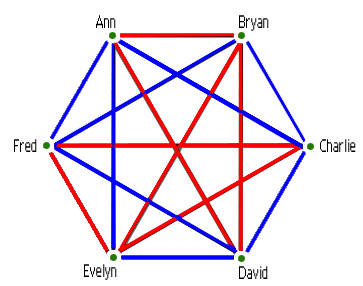This picture (called a graph) looks pretty chaotic. But you might ask if there are at least three people in the room who are either all friends (a blue triangle) or all strangers (a red triangle). In this case the answer is "yes". But what if the original graph had been different? Would we always have been able to find an orderly set of three people? In other words, would any collection of birthday guests contain three people who are all friends, or three people who are all strangers?

The answer is yes. One way of proving this would be to list all possible ways of colouring the lines in the graph in red and blue, and check each colouring for blue and red triangles. Unfortunately there are over 30,000 possible colourings, so this proof would be more than a little tedious. But there is an easier method, using a picture. First choose any point (representing a person) in your graph, and note that five lines come out of it — one to each of the other five points (representing the other five people):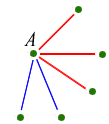With five lines there must be at least three of one colour. Let's suppose there are three red lines. So we have four points like this: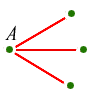What colours are the three remaining lines? If even one of them is red, then it makes a red triangle together with point A (left). And if not, the three together make a blue triangle (right):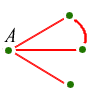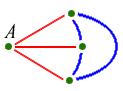We've proved that with six points (or more, of course), there must be three friends or three strangers.

### Unchartered territory

This problem, nice and neat as it is, actually leads on to questions mathematicians still haven't been able to answer. The problem suggests the question, "How many people do we need to be sure of finding either three friends, or three strangers among them?" This is our first example of a Ramsey number, and is written as R(3,3). It is named after the mathematician Frank Ramsey who was working in the 1920s. We've seen that six people is certainly enough; in other words, R(3,3) isn't any larger than 6. But would five points have been enough? The graph below with five points shows that the answer is no: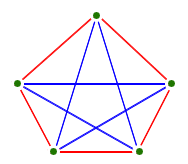So we have proved that R(3,3)=6. With a bit of work you can show that R(4,4)=18. In other words, you need 18 people to be sure there are either four friends or four strangers among them.

What about R(a,b) for general values of a and b? Can we be sure it even exists? Perhaps no number of people is enough to guarantee a friends and b strangers. But luckily we can be sure — this result is known as Ramsey's theorem. It tells us that however much orderliness we want, we can find it as long as the graph we are given is big enough.

So what is the value of R(5,5)? The answer is: nobody knows! Very few Ramsey numbers R(a,b) are actually known (with a and b both bigger than 2). The most we can say about R(5,5) with our present knowledge is that it is somewhere between 42 and 49.

How can it be so difficult to get an accurate value? Arguments involve finding upper bounds — for example, we saw quite easily that R(3,3) could not be bigger than 6. But to show that it could not be as small as 5, we had to construct a graph with 5 points, as a counterexample. The problem is that we are looking for examples of order, so the best counterexamples will usually have lots of disorder — they will look random. This makes it hard or impossible to find a "rule" that gives good counterexamples. Anything constructed by rule will probably have too much order in it.

Also, our upper bounds may be too high, but how will we ever prove it? Perhaps by examining all possible graphs on a computer to show that one has the right number of friends or strangers? The problem with this approach is that numbers explode rapidly. To show that R(5,5) is at most 49 we would have to look at 21176 possible colourings of a graph. This number is far, far bigger than the number of particles in the known Universe. So there is no chance of even the fastest computer imaginable ever finishing such a search. This is a puzzle we may never know the answer to.

This is an edited version of an article by Imre Leader which first appeared on Plus Magazine.